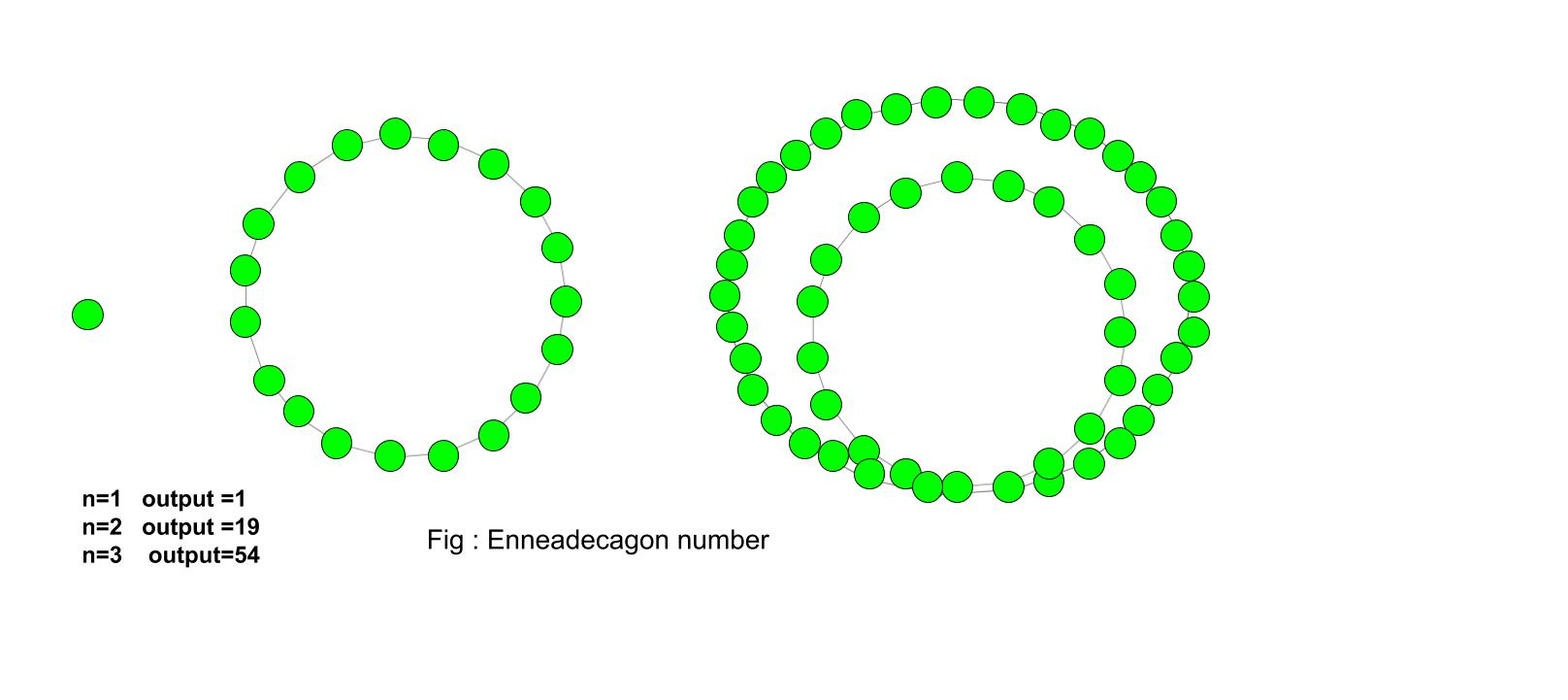Related Articles

• Last Updated : 20 Jul, 2021

Given a number n, the task is to find the nth Enneadecagonal number.
An Enneadecagonal number is a nineteen-sided polygon in mathematics. It belongs to a class of figurative numbers. The number contains the number of dots and the dots are arranged in a pattern or series. An Enneadecagonal number is also known as nonadecagon. The dots have common points and all other dots are arranged in the successive layer.

Examples :

Input : 4
Output :106
Input :10
Output :775Formula to find nth Enneadecagonal number :## C++

 // C++ program to find// nth Enneadecagonal number#include using namespace std; // Function to calculate// Enneadecagonal numberint nthEnneadecagonal(long int n){    // Formula for finding    // nth Enneadecagonal number    return (17 * n * n - 15 * n) / 2;} // Drivers codeint main(){    long int n = 6;    cout << n << "th Enneadecagonal number :" << nthEnneadecagonal(n);    return 0;}

## Java

 // Java program to find// nth Enneadecagonal numberimport java.io.*; class GFG {     // Function to calculate    // Enneadecagonal number    static int nthEnneadecagonal(int n)    {                 // Formula for finding        // nth Enneadecagonal number        return (17 * n * n - 15 * n) / 2;    }         // Driver Code    public static void main (String[] args)    {                 int n = 6;        System.out.print(n + "th Enneadecagonal number :");             System.out.println( nthEnneadecagonal(n));    }} // This code is contributed by m_kit.

## Python3

 # Program to find nth# Enneadecagonal number def nthEnneadecagonal(n) :         # Formula to calculate nth    # Enneadecagonal number    return (17 * n * n - 15 * n) // 2 # Driver Codeif __name__ == '__main__' :             n = 6    print(n,"th Enneadecagonal number :"                , nthEnneadecagonal(n)) # This code is contributed  by Ajit

## C#

 // C# program to find// nth Enneadecagonal numberusing System; class GFG{    // Function to calculate    // Enneadecagonal number    static int nthEnneadecagonal(int n)    {             // Formula for finding    // nth Enneadecagonal number    return (17 * n * n - 15 * n) / 2;    }         // Driver Code    static public void Main ()    {    int n = 6;    Console.Write(n + "th Enneadecagonal number :");         Console.WriteLine( nthEnneadecagonal(n));    }} // This code is contributed by aj_36

## PHP

 

## Javascript

 

Output:

6th Enneadecagonal number :261

Time Complexity: O(1)
Auxiliary Space: O(1)

Attention reader! Don’t stop learning now. Get hold of all the important DSA concepts with the DSA Self Paced Course at a student-friendly price and become industry ready.  To complete your preparation from learning a language to DS Algo and many more,  please refer Complete Interview Preparation Course.

In case you wish to attend live classes with experts, please refer DSA Live Classes for Working Professionals and Competitive Programming Live for Students.

My Personal Notes arrow_drop_up